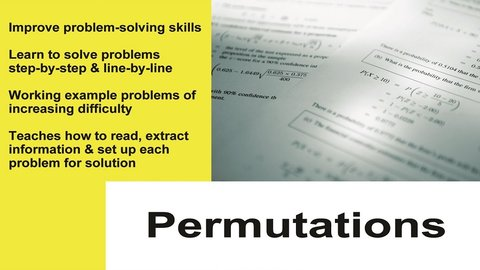Permutations Part of the Series: Probability and Statistics Tutor Series

TMW MediaPermutation as an order could also mean as a arrangement of things, sometimes the word order connotes consecutive order of things.

Related videos

Algebra
The 18 videos in this collection focus on all aspects of Algebra, from logarithms to quadratic equations and partial fractions.
Probability and Statistics Tutor Series
The Probability and Statistics Tutor is a complete 14 lesson series covering all of the core topics in detail. What sets this series apart from other teaching tools is that the concepts are taught entirely through step-by-step example problems of increasing difficulty. It works by introducing each new concept in…
Combinations
This program covers the important topic of combinations in Probability and Statistics. We begin by discussing what a combination is and why it is a central topic in probability. Next, we solve several problems that involve combinations to give students practice with the material.
Fundamentals of Probability
This program covers the important topic the fundamentals of probability in Probability and Statistics. We begin by discussing what the concept of probability really means and why it is important. Next, we solve several problems that involve the essential ideas of probability to give students practice with the material.
This program covers the important topic of the addition rules of probability in Probability and Statistics. We begin by discussing what the addition rules of probability really mean and why they are important. Next, we solve several problems that involve the essential ideas of addition in probability to give students…
Conditional Probability
This program covers the important topic conditional probability in Probability and Statistics. We begin by discussing what conditional probability is and why it is important. Next, we solve several problems that involve the essential ideas of conditional probability to give students practice with the material.
Bayes' Theorem
This program covers the important topic Bayes' Theorem in Probability and Statistics. We begin by discussing what Bayes' Theorem is and why it is important. Next, we solve several problems that involve the essential ideas of Bayes' Theorem to give students practice with the material.
Mathematical Expectation
This program covers the important topic of Mathematical Expectation in Probability and Statistics. We begin by discussing what Mathematical Expectation is and why it is important. Next, we solve several problems that involve the Mathematical Expectation to give students practice with the material.
Mean, Median and Mode
This program covers the important topic of Mean, Median, and Mode in Probability and Statistics. We begin by discussing what mean, median, and mode is and why it is important. Next, we solve several problems that involve these concepts to give students practice with the material.
Standard Deviation and Variance
This program covers the important topic of Standard Deviation and Variance in Probability and Statistics. We begin by discussing what Standard Deviation and Variance is and why it is important. Next, we solve several problems that involve these concepts to give students practice with the material.
Random Variables and Introduction to Probability Distributions
This program covers the important topic of Random Variables and Probability Distributions in Probability and Statistics. We begin by discussing what a random variable is and how it applies to probability distributions.
Binomial Probability Distribution
This program covers the important topic of the Binomial Probability Distribution in Probability and Statistics. We begin by discussing what the Binomial Distribution is and why it is important. Next, we solve several problems that involve the Binomial Distribution to give students practice with the material.